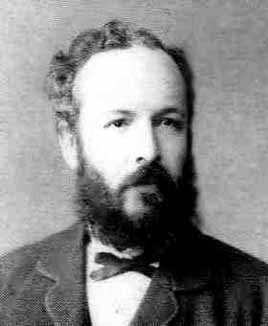Georg Ferdinand Ludwig Philipp Cantor (1845 - 1918) was a German mathematician. He is perhaps best known as the creator of set theory, which has become a fundamental theory in mathematics. Cantor established the importance of one-to-one correspondence between sets, defined infinite and well-ordered sets, and proved that the real numbers are "more numerous" than the natural numbers. (see Countable sets and Uncountable sets). In fact, Cantor's theorem implies the existence of an "infinity of infinities". He defined the cardinal and ordinal numbers, and their arithmetic.

Mathematicians call Infinite numbers "Transfinite numbers" (well they would, wouldn't they?)

Cantor formulated the Continuum Hypothesis, and defined the Cantor Set.

There is a podcast by Marcus du Sautoy, accessible from this page:

One of the Famous People on this site.
ContinuumHypothesis
RationalNumber
ZornsLemma
(none) (none)
CantorSet
CountableSet
TransfiniteNumbers
UncountableSet
(none)

## You are here

GeorgCantor
ContinuumHypothesis
FamousPeople
NaturalNumber
RealNumber
Denominator
Numerator
SquareNumber
Axiom
CountingNumber
Integer
RationalNumber
AlgebraicNumber
CategoryMetaTopic
CauchySequence
ContinuedFraction
DedekindCut
IrrationalNumber
TranscendentalNumber
WholeNumber